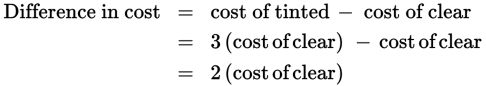# SAT Math Multiple Choice Question 445: Answer and Explanation

### Test Information

Question: 445

10. An optician charges \$125 for an eye examination, frames, and clear glass lenses, but \$197 for an eye examination, frames, and tinted glass lenses. If the tinted lenses cost three times as much as the clear lenses, how much do the clear glass lenses cost?

• A. \$24
• B. \$36
• C. \$48
• D. \$72

Explanation:

B

Difficulty: Hard

Category: Problem Solving and Data Analysis / Rates, Ratios, Proportions, and Percentages

Strategic Advice: The trick to answering a question like this is figuring out what accounts for the difference in the prices.

Getting to the Answer: In each case, the examination and the frames are the same; the difference in the cost must be due to the difference in the cost of the lenses. Because tinted lenses cost three times as much as clear lenses, the difference in cost must be twice the cost of the clear lenses.The difference in cost is \$197 – \$125 = \$72. Because this is twice the cost of the clear lenses, the clear lenses must cost \$72 ÷ 2 = \$36.